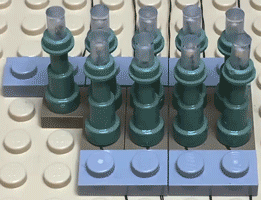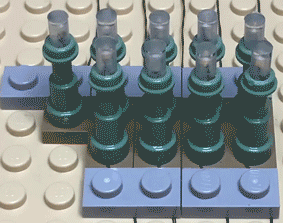# NaMaLiCS Tutorial Part 8, H_Flicker_OnOff

YAY!  This is the last of the NaMaLiCS tutorials.

Now that the core control of the system is working, loop(), and there is a template for pixel effects, cranking this out was easy.  It's just the fade effect with random flickering thrown in.

This is the core code for the fadeOn effect.  You look at the current brightness of each pixel and if it's not at maximum brightness, you increase the brightness value by 1.  You loop through this until each pixel is at the desired brightness value.

```    if( pixelNumber == 0 && pixel01 < preferredBrightness) pixel01++;
if( pixelNumber == 1 && pixel02 < preferredBrightness) pixel02++;
if( pixelNumber == 2 && pixel03 < preferredBrightness) pixel03++;```

This is the core code for the flickerOn effect.  The key differences are:

1) the brightness of the pixel is determined by multiplying the maximum brightness by a random number between 0 and 1.  Each time you loop through this code, the pixel brightness is given an arbitrary value between the minimum  and maximum brightness.

2) when checking to see if the pixel is at maximum brightness there is a little leeway (preferredBrightness - 3).  This means the randomly generated brightness doesn't need to exactly equal the maximum brightness value for the flickering to stop, it just needs to be close.  The value of 3 was determined by iterating the code until the effect "felt" right.

```    // Check each pixel in the triplet to see if it's close to the maximum
//  brightness. If it is, then stop the effect and set the brightness
//  to the maximum brightness.
if( (pixelNumber == 0) && (pixel01 < (preferredBrightness - 3)) )
// Multiply the maximum brightness by a random number between 0 and 1
//  This produces the flicker effect as the brightness jumps between
//  random values
pixel01 = (random(100) / 100.0) * preferredBrightness;
else if ( (pixelNumber == 0) && (pixel01 >= (preferredBrightness - 3)) )
pixel01 = preferredBrightness;

if( (pixelNumber == 1) && (pixel02 < (preferredBrightness - 3)) )
pixel02 = (random(100) / 100.0) * preferredBrightness;
else if ( (pixelNumber == 1) && (pixel02 >= (preferredBrightness - 3)) )
pixel02 = preferredBrightness;

if( (pixelNumber == 2) && (pixel03 < (preferredBrightness - 3)) )
pixel03 = (random(100) / 100.0) * preferredBrightness;
else if ( (pixelNumber == 2) && (pixel03 >= (preferredBrightness - 3)) )
pixel03 = preferredBrightness;```

And this is what it looks like.Because the brightness values are generated on-the-fly, no flicker effect on an individual pixel looks like the flicker effect on a different pixel.

Here is the full code for the flickerOn and flickerOff effect.  As with the fade effect, I added a slight delay, delay(2), so the effect didn't occur too quickly you couldn't see it.  Again, I will need to change this to a timing loop to get rid of the blocking a delay causes.

```// -------- FLICKER ON --------------

void flickerOn( uint8_t plugIdentifier, unsigned long delayEffectStart ) {

// Calculate the equivalent NeoPixel triplet the plug is in
uint8_t pixelSetNumber = (plugIdentifier - 1) / 3;

// Calculate the equivalent LED in the NeoPixel triplet the plug
//  corresponds to
uint8_t pixelNumber = (plugIdentifier - 1) % 3;

// Get the current brightness of the pixels in the triplet
uint32_t color = pixels.getPixelColor( pixelSetNumber );

// Get the individual brightness values of the three pixels in the triplet
uint8_t pixel01 = (uint8_t)( (color >> 16) & 0xff ),
pixel02 = (uint8_t)( (color >> 8) & 0xff ),
pixel03 = (uint8_t)( color & 0xff) ;

// Check to see if enough time has elapsed to start the flicker effect
if( currentMillis - startMillis >= delayEffectStart ) {

// Check each pixel in the triplet to see if it's close to the maximum
//  brightness. If it is, then stop the effect and set the brightness
//  to the maximum brightness.
if( (pixelNumber == 0) && (pixel01 < (preferredBrightness - 3)) )
// Multiply the maximum brightness by a random number between 0 and 1
//  This produces the flicker effect as the brightness jumps between
//  random values
pixel01 = (random(100) / 100.0) * preferredBrightness;
else if ( (pixelNumber == 0) && (pixel01 >= (preferredBrightness - 3)) )
pixel01 = preferredBrightness;

if( (pixelNumber == 1) && (pixel02 < (preferredBrightness - 3)) )
pixel02 = (random(100) / 100.0) * preferredBrightness;
else if ( (pixelNumber == 1) && (pixel02 >= (preferredBrightness - 3)) )
pixel02 = preferredBrightness;

if( (pixelNumber == 2) && (pixel03 < (preferredBrightness - 3)) )
pixel03 = (random(100) / 100.0) * preferredBrightness;
else if ( (pixelNumber == 2) && (pixel03 >= (preferredBrightness - 3)) )
pixel03 = preferredBrightness;
}

// Update the pixel triplet with the new values
color = pixels.Color( pixel01, pixel02, pixel03 );
pixels.setPixelColor( pixelSetNumber, color );

// Add a slight delay so the fade effect doesn't happen to quickly
delay(2);
}

// -------- FLICKER OFF --------------

void flickerOff( uint8_t plugIdentifier, unsigned long delayEffectStart ) {

// Calculate the equivalent NeoPixel triplet the plug is in
uint8_t pixelSetNumber = (plugIdentifier - 1) / 3;

// Calculate the equivalent LED in the NeoPixel triplet the plug
//  corresponds to
uint8_t pixelNumber = (plugIdentifier - 1) % 3;

// Get the current brightness of the pixels in the triplet
uint32_t color = pixels.getPixelColor( pixelSetNumber );

// Get the individual brightness values of the three pixels in the triplet
uint8_t pixel01 = (uint8_t)( (color >> 16) & 0xff ),
pixel02 = (uint8_t)( (color >> 8) & 0xff ),
pixel03 = (uint8_t)( color & 0xff) ;

// Check to see if enough time has elapsed to start the flicker effect
if( currentMillis - startMillis >= delayEffectStart ) {

// Check each pixel in the triplet to see if it's close to the minimum
//  brightness. If it is, then stop the effect and set the brightness
//  to minimum.
if( (pixelNumber == 0) && (pixel01 > (minBrightness + 3)) )
pixel01 = (random(100) / 100.0) * preferredBrightness;
else if ( (pixelNumber == 0) && (pixel01 <= (minBrightness + 3)) )
pixel01 = 0;
if( (pixelNumber == 1) && (pixel02 > (minBrightness + 3)) )
pixel02 = (random(100) / 100.0) * preferredBrightness;
else if ( (pixelNumber == 1) && (pixel02 <= (minBrightness + 3)) )
pixel02 = 0;
if( (pixelNumber == 2) && (pixel03 > (minBrightness + 3)) )
pixel03 = (random(100) / 100.0) * preferredBrightness;
else if ( (pixelNumber == 2) && (pixel03 <= (minBrightness + 3)) )
pixel03 = 0;
}

// Update the pixel triplet with the new values
color = pixels.Color( pixel01, pixel02, pixel03 );
pixels.setPixelColor( pixelSetNumber, color );

// Add a slight delay so the fade effect doesn't happen to quickly
delay(2);
}```

And this is the full suite of pixel effects that will be used in my National Mall project.  I know, tough to tell the difference between the blink effect and the sequential flicker effects, but the effects were sped up so the posted video wouldn't be huge.  Each effect will occur more slowly IRL.And that it!

If you have any interest, questions, suggestions or comments, post'em here or PM me.

As for me, next on the list is getting the whole system working with protothreads, figuring out how best to scale this up from 9 light to ~1,200 lights, and actually installing the BrickPixel hardware and lights in the National Mall.  Hopefully more interesting videos will be posted soon.

See ya!

-wrtyler-

Many thanks to Josh here on the forum for pointing me to protothreads and how to implement them and to Rob Klingberg  & Brickstuff for making an incredible light control system, BrickPixel, and providing much needed help, advice and information as worked my way through this.

I hope, when this project is finished, it will be a showcase for what you can do with the BrickPixel system.  I'm looking to have it ready for Bricks Cascade in Portland, March 10th - 13th, 2022.  Hope to see you there.  Come over and say hi.

2replies Oldest first
• Oldest first